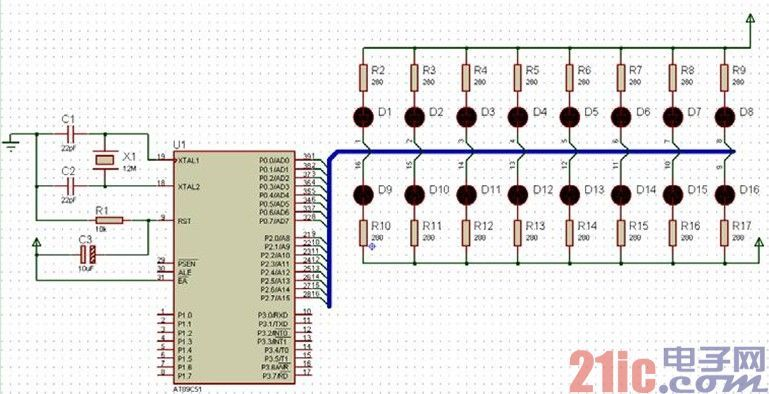# 单片机C语言编程：TIMER0 控制流水灯

TIMER0 单片机 C语言编程 控制流 作者：admin 来源:不详 发布时间：2018-05-18 20:56:36   浏览：21

/*  名称：TIMER0 控制流水灯

*/

#include<reg51.h>

#include<intrins.h>

#define uchar unsigned char

#define uint unsigned int

//主程序

void main()

{

uchar T_Count=0;

P0=0xfe;

P2=0xfe;

TMOD=0x01; //定时器 0 工作方式 1

TH0=(65536-40000)/256; //40ms 定时

TL0=(65536-40000)%256;

TR0=1;         //启动定时器

while(1)

{

if(TF0==1)

TF0=0;

TH0=(65536-40000)/256; //恢复初值

TL0=(65536-40000)%256;

if(++T_Count==5)

{

P0=_crol_(P0,1);

P2=_crol_(P2,1);

T_Count=0;

}

}

}

}